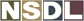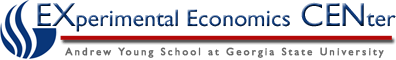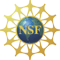Students / Subjects# Preferences

For any two alternative consumption points x and x' that are both within the consumer's budget set, a choice of x rather than x' indicates a preference for x relative to x', which is written x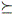x'. Since x and x' may be equally good from the perspective of this consumer, xx' means that "the consumer likes x at least as much as she likes x'."

If we make several assumptions about properties of preferences, then we will be able later to represent preferences in a convenient way, called a utility function. Most of these properties are fairly natural, especially when the number of alternatives is small.

### Properties of Preferences

There are several properties of preferences that together imply that a consumer's choices will be consistent.

### P.1 Preferences are complete

Preferences are complete if for any two consumption points x and x', either xx' (x is at least as good as x') or x'x (x' is at least as good as x), or both.

For example, x may be one apple and one mango, and x' might be one orange and one carrot. The property says that when presented with these two alternatives, I can decide that "x is at least as good as x'" or "x' is at least as good as x." While this appears like a very mild assumption, completeness asserts that I can do this for every possible pair of choices in the set of alternatives, which may be a demanding task.

### P.2 Preferences are reflexive

Preferences are reflexive if for all x, xx (x is at least as good as itself).

This assumption is probably the weakest of the five assumptions. In the example above, it would assert that "I like one apple and one mango at least as well as one apple and one mango."

### P.3 Preferences are transitive

Preferences are transitive if xx' and x'x'' implies that xx''.

Transitivity is also intuitive and plausible. If x and x are the same two alternatives as in the example of P.1, and x'' is one banana and one carrot, then transitivity would assert that if "I like one apple and one mango at least as well as one orange and one carrot" and ``I like one orange and one carrot at least as well as one banana and one carrot" then "I like one apple and one mango at least as well as one banana and one carrot."

### P.4 Preferences are strongly monotonic

Preferences are strongly monotonic if for any two commodity points x = (x1, x2) and x' = (x'1, x'2) if x1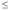x'1, x2x'2, and x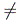x', then x' is preferred to x.

Strong monotonicity means that for a specified consumption level x, there is some point x' close to x that is preferred to x. The point x' might have more of both commodities, or it may only have more of one commodity.

Go to Derived Properties of Preferences.